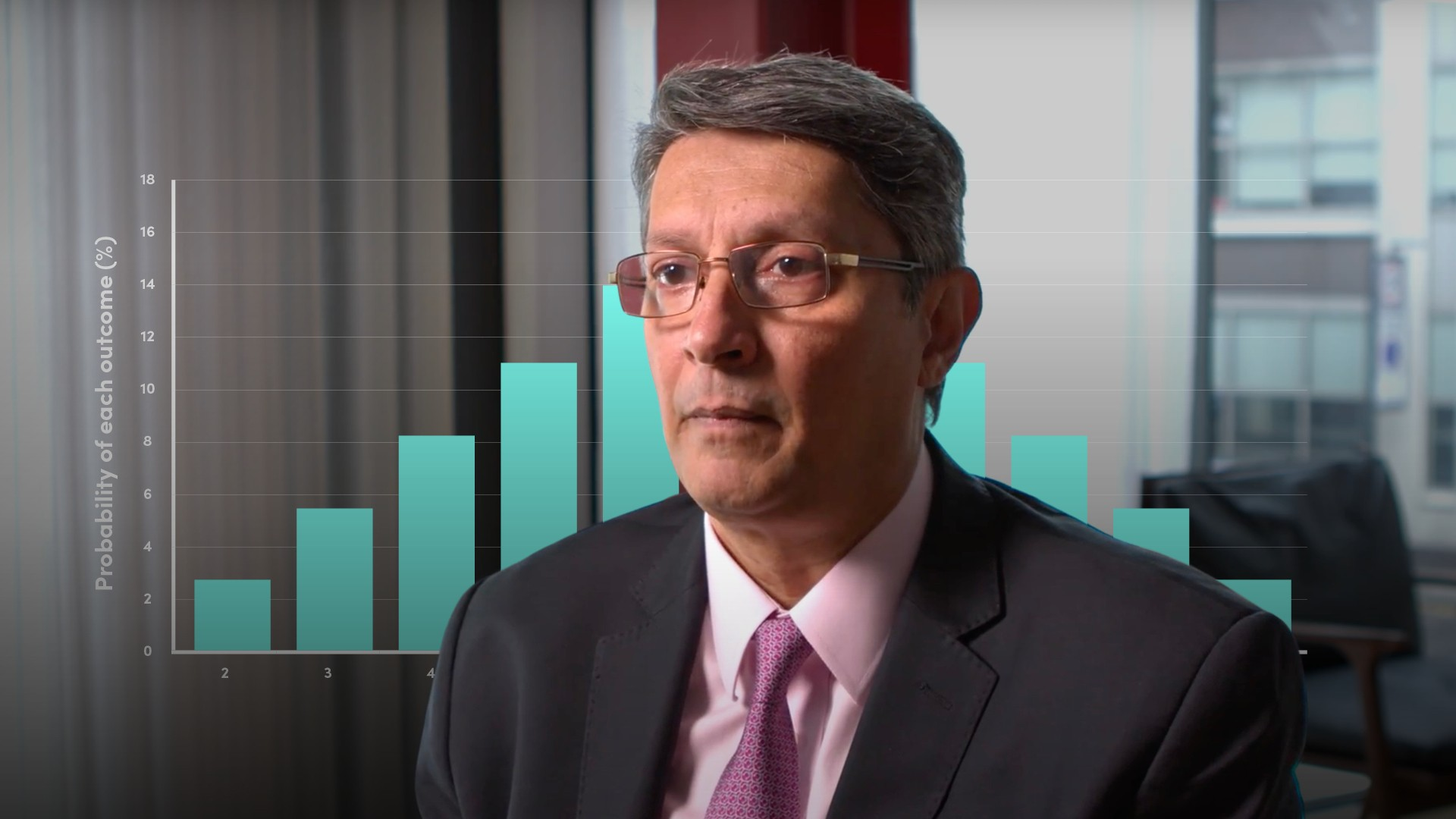# Probability Distributions (2/7): Cumulative Distribution Function### Abdulla Javeri

In the previous video of the series, Abdulla introduced the concept of probability distributions. In this video, Abdulla will expand and explore the cumulative distribution function. He explains how to calculate it and how to use it by building on the example featured in the video on the basics of probability.

Financial Modelling & Mathematics